Free Shipping for Online Orders. Terms and conditions apply.

## Rotary Device Sizing Tool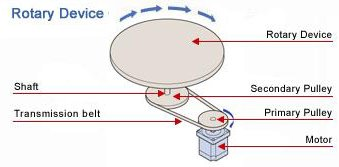Select the unit

### Table shape and dimensions

Round Table

Rectangular table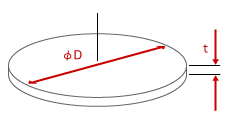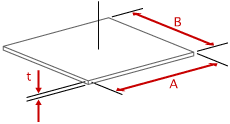Table diameter

D = in mm

A = in mm

B = in mm

Table weight mass

W m = lb kg

If you are not sure about the weight mass

Table thickness

t = in mm

Table Material

ρ =

### Drive shaft dimension

Shaft diameter

D2 = in mm

Shaft weight mass

W2 m2 = lb kg

If you are not sure about the weight mass

Length

L = in mm

Material

ρ2 =

 No additional load Cylinder type Rectangular pillar type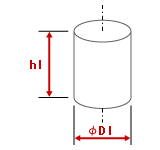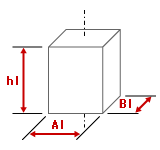D1 = in mm

A1 = in mm

B1 = in mm

Distance from the table center to the load center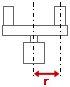r = in mm

n = pc

W1 m1 = lb/pc kg/pc

If you are not sure about the weight mass

h1 = in mm

ρ1 =

### Table support (Leave the fields blank if the friction coefficient can be ignored)

Friction coefficient between the table and the supporting mechanism

μ=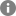Distance from the table center to the supporting mechanism
(Please specify the diameter if you use Ball bearing)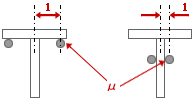l = in mm

System efficiency

η= %

### Transmission belt and pulleys or gears (Leave the fields blank if a direct coupling structure is used)

Primary pulley (gear) pitch circle diameter (PCD) or diameter

Secondary pulley (gear) pitch circle diameter (PCD) or diameter

Dp1

=   in mm

Dp2

=   in mm

Primary pulley (gear) weight mass

Secondary pulley (gear) weight mass

Wp1 mp1

=   lb kg

Wp2 mp2

=   lb kg

If you are not sure about the weight mass

If you are not sure about the weight mass

Primary pulley (gear) thickness

Secondary pulley (gear) thickness

Lp1

=   in mm

Lp2

=   in mm

Primary pulley (gear) material

Secondary pulley (gear) material

ρp1

=

ρp2

=

### Mechanism condition

Horizontal operation

Vertical operation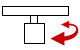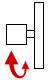### Other requirement(s)

It is necessary to hold the load even after the power supply is turned off.
→ You need an electromagnetic brake.

It is necessary to hold the load after the motor is stopped, but not necessary to hold after the power supply is turned off.

### Operating conditions

 Fixed speed operation Operating speed V1 = r/min Acceleration/Deceleration t1 = s
 Variable speed operation Operating speed V1 = r/min 〜 V2 = r/min Acceleration/Deceleration t1 = s
 Positioning operation (Fill in the fields, if any)Rotor inertia JO = oz·in kg·m 2 Gear ratio i = If the rotor inertia and the gear ratio are unknown, the acceleration torque will be calculated with an inertia ratio of 5:1 (see the motor selection tips that will appear on the result window for the detail).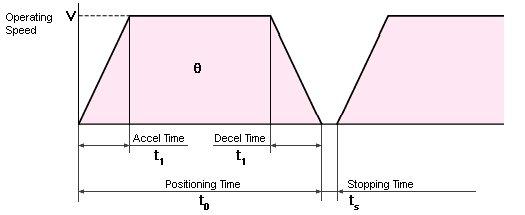Positioning distance θ = ° Positioning time t0 = s Stopping time ts = s If a specific acceleration / deceleration time is required t1 = s If a specific operating speed is required V = r/min If Positioning distance is given and acceleration/deceleration is unknown, it is calculated as one fourth of Positioning time.

### Stopping accuracy

Stopping accuracy

±

Δθ

=

°

or

±

Δl

=

in mm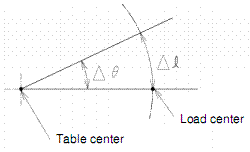### Safety factor

Safety factor

The following is the estimated requirements. Please contact 1-800-468-3982 ( from overseas 1-847-871-5931 ) for assistance or questions.

### Sizing Results

JL

= [oz·in [kg·m 2]

Required Speed

V1

= [r/min]

V2

= [r/min]

Required Torque

T

= [lb·in] = [oz·in] [N·m]

RMS Torque

Trms

= [lb·in] = [oz·in] [N·m]

Acceleration Torque

Ta

= [lb·in] = [oz·in] [N·m]

TL

= [lb·in] = [oz·in] [N·m]

Required Stopping Accuracy

Δθ

= [deg]

Other Requirement(s)

To print the calculation report, click    Full Report
To view the motor selection tips, click    Tips

×Call 1-800-GO-VEXTA(468-3982) or 1-847-871-5931Print

- given information -

### Table shape and dimensions

Table type

Diameter

D =  [in] [mm]

Width

A =  [in] [mm]

Depth

B =  [in] [mm]

Weight Mass

W m [lb] [kg]

Thickness

t =  [in] [mm]

Material

ρ =  [oz/in [kg/m 3]

### Drive shaft dimension

Shaft diameter

D2 [in] [mm]

Shaft weight mass

W2 m2 [lb] [kg]

Shaft length

L =  [in] [mm]

Shaft material

ρ2 [oz/in [kg/m 3]

Diameter

D1 [in] [mm]

Width

A1 [in] [mm]

Depth

B1 [in] [mm]

Distance from the table center to the load center

r =  [in] [mm]

n =  pc

W1 m1 [lb] [kg]

h1 [in] [mm]

ρ1 [oz/in [kg/m 3]

### Table support

Friction coefficient between the table and the supporting mechanism

μ =

Distance from the table center to the supporting mechanism

l =  [in] [mm]

System efficiency

η =  %

### Transmission belt and pulleys or gears

Primary pulley (gear)

Secondary pulley (gear)

pitch circle diameter (PCD)

Dp1

= [in] [mm]

Dp2

= [in] [mm]

weight mass

Wp1 mp1

= [lb] [kg]

Wp2 mp2

= [lb] [kg]

thickness

Lp1

= [in] [mm]

Lp2

= [in] [mm]

material

ρp1

= [oz/in [kg/m 3]

ρp2

= [oz/in [kg/m 3]

### Mechanism Condition

Mechanism Condition

### Other requirement(s)

Is it necessary to hold the load even after the power supply is turned off?

Is it necessary to hold the load after the motor is stopped, but not necessary to hold after the power supply is turned off?

### Operating conditions

Fixed speed operation

Operating speed

V1

=

[r/min]

Acceleration / deceleration time

t1

=

[s]

### Operating conditions

Variable speed operation

Operating speed

V1

=

[r/min]

V2

=

[r/min]

Acceleration / deceleration time

t1

=

[s]

### Operating conditions

Positioning operation

Rotor inertia

JO

=

[oz·in kg·m 2]

Gear ratio

i

=

Positioning distance

θ

=

°

Positioning time

t0

=

[s]

Stopping time

ts

=

[s]

Acceleration / deceleration time

t1

=

[s]

Specified speed

V

=

[r/min]

### Stopping accuracy

Stopping accuracy

± Δθ

=  °

± Δl

= [in] [mm]

### Safety factor

Safety factor

S·F

=

- calculated result -

Jt

=   (1/8) (w × 16 ) × D2 (π/32) ρ t × D4 (1/12) (w × 16) (A2 + B2) (1/12) p A B t (A2 + B2) (1/8) m × (D × 10-3)2 (π/32) ρ (t × 10-3 ) × (D × 10-3)4 (1/12) m ( (A × 10-3)2 + (B × 10-3)2) (1/12) ρ (A × 10-3) (B × 10-3) (t × 10-3 ) ( (A × 10-3 )2 + (B × 10-3 )2)

=   (1/8) ( × 16 ) × 2 (3.4/32) × × 4 (1/12) ( × 16 ) × ( 2 + 2 ) (1/12) × × × × ( 2 + 2 ) (1/8) × × ( × 10-3)2 (3.4/32) × ( × 10-3) × ( × 10-3)4 (1/12) × ((× 10-3)2 + (× 10-3)2 ) (1/12) × × ( × 10-3) × ( × 10-3) × ( × 10-3) (( × 10-3)2 + ( × 10-3)2 )

= [oz·in [kg·m 2]

JS

=   (π/32) ρ2 L D24 (1/8) (W2 × 16) × D22 (π/32) ρ (L × 10-3) (D2 × 10-3)4 (1/8) m2 (D2 × 10-3)2

=   ( 3.14 / 32 ) × × × 4 (1/8) × ( × 16 ) × 2 =   ( 3.14 / 32 ) × × ( × 10-3) × ( × 10-3)4 (1/8) × × ( × 10-3)2

= [oz·in [kg·m 2]

J1

=   ((1/8) (W1 × 16) × D12 + (W1 × 16) r2) × n ((π/32) ρ h1 D14 + (π/4) ρ h1 D12 r2) × n (1/12) (W1 × 16) × (A12 + B12 + 12 × r2) × n (1/12) (ρ A1 B1 h1 (A12 + B12 + 12 × r2) × n ((1/8) m1( D1 ×10-3)2 + m1 (r ×10-3)2) × n ((π/32) ρ (h1 ×10-3) (D1 ×10-3)4 + (π/4) ρ (h1 ×10-3) (D1 ×10-3)2 (r ×10-3)2 ) × n (1/12) m1 ((A1 ×10-3)2 + (B1 ×10-3)2 + 12 × (r ×10-3)2) × n (1/12) ρ (A1 ×10-3) (B1 ×10-3) (h1 ×10-3) ((A1 ×10-3)2 + (B1 ×10-3)2 + 12 × (r ×10-3)2) × n

=   ((1/8) × ( × 16) × 2 + ( × 16) × 2) × ((3.14/32) × × × 4 + (3.14/4) × × 2 × 2) × (1/12) ( × 16) × ( 2 + × 2 + 12 × 2) × (1/12) ( × × × ) × ( 2 + 2 + 12 × 2) × ((1/8) × × ( ×10-3)2 + ( × 16) × ( ×10-3)2) × ((3.14/32) × × ( ×10-3) × ( ×10-3)4 + (3.14/4) × ( ×10-3) × ( ×10-3)2 × ( ×10-3)2) × (1/12) × × (( ×10-3)2 + × ( ×10-3)2 + 12 × ( ×10-3)2) × (1/12) × × ( ×10-3) × ( ×10-3) × ( ×10-3) × (( ×10-3)2 + ( ×10-3)2 + 12 × ( ×10-3)2) ×

= [oz·in [kg·m 2]

JDp1

=  ( 1 / 8 ) wp1 × 16 × Dp1 mp1 × (Dp1×10-3) 2

=   ( 1 / 8 ) ×  × 16 × ( ×10-3) 2

= [oz·in [kg·m 2]

JDp1

=   ( π / 32 ) ρp1 ( Lp1 ×10-3) ( Dp1 ×10-3) 4

=   ( 3.14 / 32 ) ×  × ( ×10-3)  × ( ×10-3) 4

= [oz·in [kg·m 2]

JDp2

=   ( 1 / 8 ) wp2 × 16 × Dp2 mP2 × (DP2×10-3) 2

=   ( 1 / 8 ) ×  × 16 × ( ×10-3) 2

= [oz·in [kg·m 2]

JDp2

=  ( π / 32 ) ρp2 ( Lp2 ×10-3) ( Dp2 ×10-3) 4

=   ( 3.14 / 32 ) ×  × ( ×10-3)  × ( ×10-3) 4

= [oz·in [kg·m 2]

JL

=   ( Jt + Js + Jl + JDp2 ) ( Dp1 / Dp2 )2 + JDp1

= (  +  +  +  ) × (  /  )2 +

[oz·in [kg·m 2]

JL

=  Jt + Js + Jl

=  (  +  + )

[oz·in [kg·m 2]

### Required Speed

 Vm =   V   ( Dp2 / Dp1 ) =     × (  /  ) = [r/min]
 Vm1 =   V1 ( Dp2 / Dp1 ) =     × (  /  ) = [r/min] Vm2 =   V2 ( Dp2 / Dp1 ) =     × (  /  ) = [r/min]
 Vm =  V × ( Dp2 / Dp1 ) =   × (  /  ) = [r/min] Vm ( θ / 360) × ( 60 / ( t0 - t1 ) ) × ( Dp2 / Dp1 ) (  / 360)  ) × (60 / ( - )) × (  /  ) = [r/min]

### Required Torque

T

=   ( Ta + TL ) ( Safety Factor )

= (  +  ) ×

= [lb·in] [N·m]

= [oz·in]

### RMS Torque

Trms

=   √(((( Ta + TL )2 × t1 ) + ( TL2 × (t0 - 2 × t1 )) + (( Ta - TL )2 × t1 )) / ( t0 + ts )) × (Safety Factor)

=   √ ((((  +  )2 ×  ) + ( 2 × (  - 2 ×  )) + ((  -  )2 ×  )) / (  +  )) ×

= [lb·in] [N·m]

= [oz·in]

### Acceleration Torque

 Ta =   ( JL / 386 ) ( Vm / ( 9.55 × t1 )) ( 1 / 16 ) = (   / 386 ) × (  / ( 9.55 ×  )) × ( 1 / 16 ) = [lb·in] [N·m] = [oz·in]
 Ta =   ( JL / 386 ) ( Vm / ( 9.55 × t1 )) ( 1 / 16 ) = (  / 386 ) × (  / ( 9.55 ×  )) × ( 1 / 16 ) = [lb·in] [N·m] = [oz·in]
 Ta =   (( 1.2 × JL ) / 386 ) × ( Vm / ( 9.55 × t1 )) (( JO + JL )/386) × ( Vm / ( 9.55 × t1 )) (( JO × i2 + JL )/386) × ( Vm / ( 9.55 × t1 )) × ( 1 / 16 ) ( 1.2 × JL ) × ( Vm / ( 9.55 × t1 )) ( JO + JL ) × ( Vm / ( 9.55 × t1 )) ( JO × i2 + JL) × ( Vm / ( 9.55 × t1 )) = (( 1.2 × / 386 ) × ( / ( 9.55 × )) × ( 1 / 16 ) (( + )/386) × ( / ( 9.55 × )) × ( 1 / 16 ) (( × 2 + )/386) × ( / ( 9.55 × )) × ( 1 / 16 ) ( 1.2 × ) × ( / ( 9.55 × ) ( + ) × ( / ( 9.55 × )) ( × 2 + ) × ( / ( 9.55 × )) = [lb·in N·m] = [oz·in]

WT mT

=   W m (1/16) (π / 4) ρ t D2 (π / 4) ρ (t ×10-3 ) (D ×10-3)2 (1/16) ρ A B t ρ (A ×10-3) (B ×10-3) (t ×10-3)

=   (1/16) (3.14 / 4) × × × 2 (3.14 / 4) × × ( ×10-3 ) × ( ×10-3)2 (1/16) × × × × × ( ×10-3) × ( ×10-3) × ( ×10-3)

[lb Kg]

W1 m1

=   No additional load w1 m1 × n (1/16) (π / 4) ρ1 h1 D12 n (π / 4) ρ1 (h1 × 10-3 ) (D1 × 10-3)2 n (1/16) ρ1 A1 B1 h1 n 1 (A1 × 10-3 ) (B1 × 10-3 ) (h1 × 10-3)) × n

=   0 × (1/16) × (3.14 / 4) × × × 2 × × (3.14 / 4) × × ( × 10-3) × ( × 10-3)2 × (1/16) × × × × × × × ( × 10-3) × ( × 10-3) × ( × 10-3) ×

= [lb Kg]

TL

=   ( WT + W1) µ 9.8 ( mT + m1) µ (l × 10-3) (1 / (η × 0.01)) ( W1 /2 ) r ( 9.8 m1 /2) (r × 10-3) (1 / (η × 0.01)) ( Dp1 / Dp2 )

=   9.8 × ( + ) × × ( × 10-3) × (1 / ( × 0.01)) ( / 2 ) × ( 9.8 × / 2) × ( × 10-3) × (1 / ( × 0.01)) × (  /  )

= [lb·in] [N·m]

=   [oz·in]

### Required Stopping Accuracy

Δθ

=   Δθ Δl ( 360° / π D ) Δl ( 360° / 2 π r ) ( Dp2 / Dp1 )

=    × ( 360 / (3.14 × )  )  × ( 360 / (2 × 3.14 × )  ) × (  /  )

[deg]

### Other requirement(s)

- end of the report -